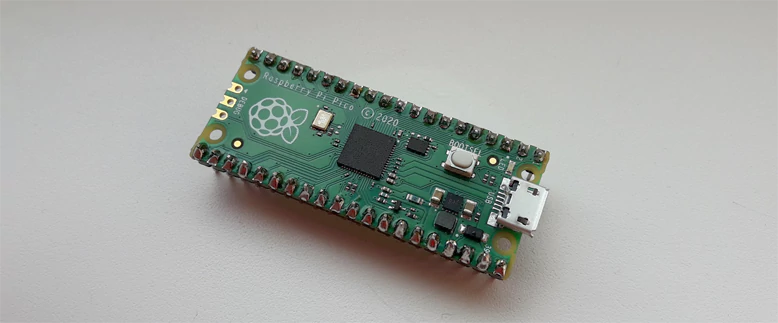# Measure Execution Time of Code using Raspberry Pi Pico

Execution time of a code is amount of time spent by the system executing that code. Execution time is important when want to optimize a code. This tutorial shows how to measure execution time of a code using Raspberry Pi Pico.

## Code

The function `clock` defined in the `time.h` header returns the number of clock ticks elapsed since the program was started. CPU clock ticks can be converted to seconds by dividing value by `CLOCKS_PER_SEC` constant. However, the `clock` function is not implemented in the Raspberry Pi Pico SDK. The `clock` function can be implemented by using `time_us_64` function provided in the SDK. It returns the current 64-bit timestamp value in microseconds.

Execution time is difference between the end time and start time. To measure execution time of a code, subtract the start time from the end time.

project/main.c

``````#include <time.h>
#include <stdio.h>
#include <pico/stdlib.h>

clock_t clock()
{
return (clock_t) time_us_64() / 10000;
}

void doSomething(int seconds)
{
sleep_ms(seconds * 1000);
}

int main()
{
stdio_init_all();

while (true) {
clock_t startTime = clock();
doSomething(1);
clock_t endTime = clock();

double executionTime = (double)(endTime - startTime) / CLOCKS_PER_SEC;
printf("%.8f sec\n", executionTime);
}
}``````

project/CMakeLists.txt

``````cmake_minimum_required(VERSION 3.13)

include(\$ENV{PICO_SDK_PATH}/external/pico_sdk_import.cmake)

project(myapp C CXX ASM)

set(CMAKE_C_STANDARD 11)
set(CMAKE_CXX_STANDARD 17)

pico_sdk_init()

Project was built and binary file `myapp.uf2` was moved to the mounted drive RPI-RP2. To see results, we use the Serial Monitor tool in the Arduino IDE. A program prints `1.00000000 sec` as expected.